# Electric Circuits, Circuit Diagram & Ohm's Law Notes | Study Science Class 10 - Class 10

## Class 10: Electric Circuits, Circuit Diagram & Ohm's Law Notes | Study Science Class 10 - Class 10

The document Electric Circuits, Circuit Diagram & Ohm's Law Notes | Study Science Class 10 - Class 10 is a part of the Class 10 Course Science Class 10.
All you need of Class 10 at this link: Class 10

Electric Circuits and Measuring Instruments

A closed path in which a current can flow is called an electric circuit. An electric circuit may have one or more electric elements such as bulbs (or lamps), cells, switches (or plug keys), metal wires, etc. Each element of a circuit has a specific function to play. For example, wires can be used to connect one element to the next. And a plug key or a switch can be used to either complete or break the closed path, thereby starting or stopping the current in the circuit.
Some common circuit elements and their symbols.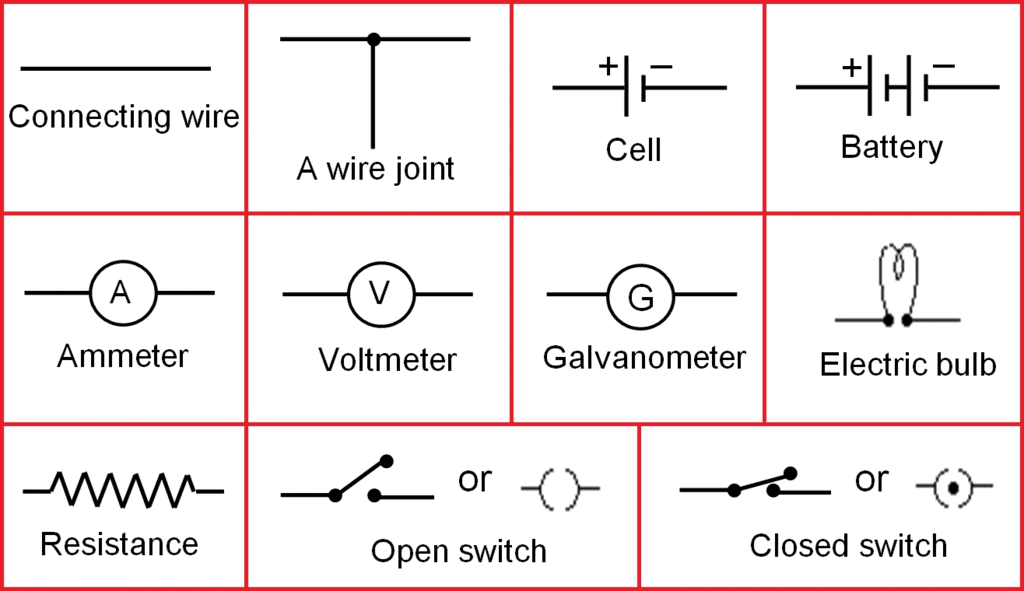Fig. Some symbols used in circuit diagrams

Common Measuring Instruments

The electric current in a circuit is measured by an instrument called the ammeter, and the potential difference between two points in it is measured by a voltmeter (in voltage stabilizers). In these meters, a needle moving over a graduated scale gives the value of the measured quantity. Each meter has two terminals. The terminal marked '+' is connected by a wire to the higher-potential side of a circuit, while the terminal marked '' is connected to the lower-potential side.

Using an Ammeter to measure Current
To measure the current through an element of a circuit, an ammeter is connected in such a way that the current flowing through it also flows through the element. Such a connection is called a series connection. In Figure, the current i flowing through the lamp also flows through the ammeter. The reading of the ammeter gives the current through the lamp. Note that if the ammeter is removed, there will be a gap, and the current through the circuit will stop.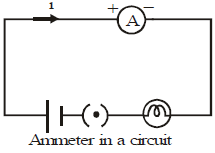Two or more electric elements are said to be connected in series if the current flowing through one also flows through the rest.
An ammeter is always connected in series in a circuit.

Using a Voltmeter to measure Potential Difference

Figure shows a circuit that has two lamps connected to a cell. We want to measure the potential difference across the lamp L2, i.e., between the points A and B. As A is on the side of the positive terminal of the cell, its potential is higher than that of B. So, the '+' terminal of the voltmeter is connected to A, and the '' terminal, to B. The reading of the voltmeter gives the potential difference across L2. The current flowing through the voltmeter is different from those flowing through the other elements of the circuit. Also, even if the voltmeter is removed, the current continues to flow in the circuit. Note that the potential difference across L2 and the voltmeter is the same. Such a connection is called a parallel connection.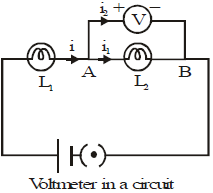Two or more electric elements are said to be connected in parallel if the same potential difference exists across them.

Ohm's Law

The electric current through a metallic element or wire is directly proportional to the potential difference applied between its ends, provided the temperature remains constant.

If a potential difference V is applied to an element and a current i passes through it,

i  ∝  V

or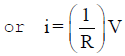Thus Ohm's Law V = iR

Here R is a constant for the given element (metallic wire) at a given temperature and is called its resistance. It is the property of a conductor to resist the flow of charges through it.

Resistance

From equation,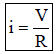So, for a given potential difference,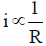Thus, for a given potential difference, the current is inversely proportional to the resistance. The higher is the resistance, the lower is the current. If the resistance is doubled, the current is halved. Good conductors have low resistance, while insulators have very high resistance.

Unit of Resistance

Potential difference is measured in volts, and current is measured in amperes. From Equation, R = V/i. So, the unit of resistance is volt/ampere. This unit is called the ohm, and its symbol is W. We can define one ohm as follows.

If a potential difference of 1 volt is applied across an element, and a current of 1 ampere passes through it, the resistance of the element is called 1 ohm.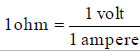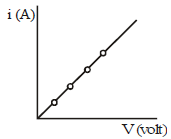Factors on which the Resistance of a Conductor Depends

The resistance of the conductor depends

(i) on itlength,

(ii) on its area of cross-section, an

(iii) on the nature of its material.

Resistance of a uniform metallic conductor is directly proportional to its length (l) and inversely proportional to the area of cross-section (A).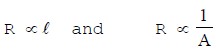Combining eqs. we get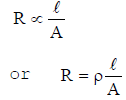Where ρ(rho) is a constant of proportionality and is called electrical resistivity of the material of the conductor. The SI unit of resistivity is Ωm.

The document Electric Circuits, Circuit Diagram & Ohm's Law Notes | Study Science Class 10 - Class 10 is a part of the Class 10 Course Science Class 10.
All you need of Class 10 at this link: Class 10Use Code STAYHOME200 and get INR 200 additional OFF

## Science Class 10

107 videos|325 docs|162 tests

Track your progress, build streaks, highlight & save important lessons and more!

,

,

,

,

,

,

,

,

,

,

,

,

,

,

,

,

,

,

,

,

,

,

,

,

;